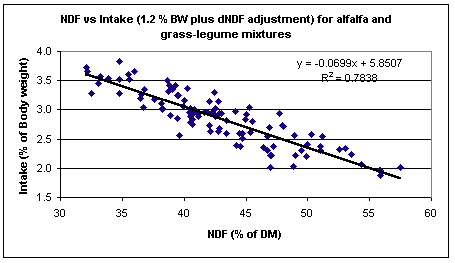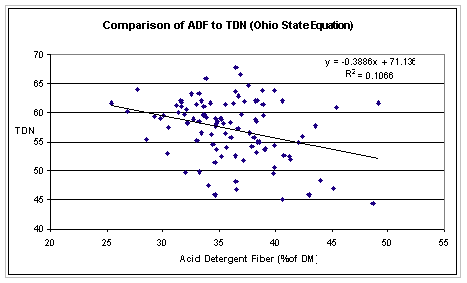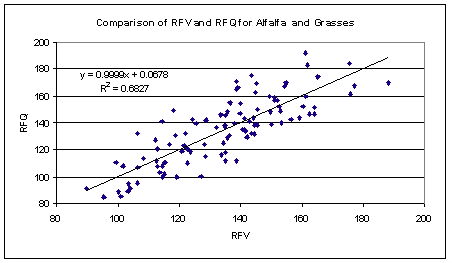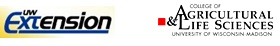# Relative Forage Quality (RFQ) – Indexing Legumes and Grasses for Forage Quality# Relative Forage Quality (RFQ) Indexing Legumes and Grasses for Forage Quality

by Dan Undersander, University of Wisconsin
and John E. Moore, University of Florida

Relative Feed Value has been of great value in ranking forages for sale or inventorying and assigning forage to animal groups according to their quality needs. With the introduction of the new approaches to determining animal requirements in National Research Council Nutrient Requirements for Dairy Cattle (2001), there is an opportunity to improve upon this quality index through use of newer analyses and equations.

Relative Feed Value was based on the concept of digestible dry matter intake relative to a standard forage according to the following:

RFV = (DMI, % of BW) × (DDM, % of DM) ÷ 1.29

Where: DMI = dry matter intake
DDM = digestible dry matter

Dry matter intake was estimated from NDF and DDM from acid detergent fiber. The constant, 1.29, was chosen so that RFV = 100 for full bloom alfalfa. The constant was the expected DDM intake, as % of BW, for full-bloom alfalfa based on animal data.

We propose to keep the same concept and format for Relative Forage Quality (RFQ) except that TDN will be used rather than DDM. Thus, RFQ will be as follow:

RFQ = (DMI, % of BW) × (TDN, % of DM) ÷ 1.23

Where the divisor, 1.23, is used to adjust the equation to have a mean and range similar to RFV (Moore and Undersander, 2002). The following two equations are recommended depending on whether the primary forage is legume or grass:

1. For alfalfa, clovers, and legume/grass mixtures the equations for TDN and DMI will be:

Total digestible nutrients for alfalfa, clover and legume/grass mixtures are calculated from the new NRC recommendations using in vitro estimates of digestible NDF as follows:

TDNlegume = (NFC × .98) + (CP × .93) + (FA × .97 × 2.25) + (NDFn ÷ 100) − 7) (NRC, 2001)

Where: CP = crude protein (% of DM)
EE = ether extract (% of DM)
FA = fatty acids (% of DM) = ether extract – 1
NDF = neutral detergent fiber (% of DM)
NDFCP = neutral detergent fiber crude protein
NDFn = nitrogen free NDF = NDF – NDFCP, else estimated as NDFn = NDF × .93
NDFD = 48-hour in vitro NDF digestibility (% of NDF)
NFC = non-fibrous carbohydrate (% of DM) = 100 – (NDFn + CP + EE + ash)

Dry matter intake calculations for alfalfa, clover and legume/grass mixtures will be:

DMILegume = 120 ÷ NDF + (NDFD − 45) × .375 ÷ 1350 × 100 (Mertens, 1987 with NDFD adjustment by Oba and Allen (1999).  45 is an average value for fiber digestibility of alfalfa and alfalfa/grass mixtures.)

Where DMI is expressed as % of body weight (BW), NDF as % of DM and NDFD as % of NDF.

RFQ = (DMIlegume, % of BW) × (TDNlegume, % of DM) ÷ 1.23

spa2.   For warm and cool season grasses the equations for TDN and DMI will be:

Total digestible nutrients for warm and cool season grasses are calculated as:

TDNgrass = (NFC × .98) + (CP × .87) + (FA × .97 × 2.25) + (NDFn × NDFDp ÷ 100) − 10 (Moore and Undersander, 2002)

Where terms are as defined previously and
spacesNDFDp = 22.7 + .664 × NDFD

Dry matter intake calculations for warm and cool season grasses will be:

DMIGrass = -2.318 + 0.442 × CP − 0.01 × CP2 − 0.0638 × TDN + 0.000922 × TDN2 + 0.18 × ADF − 0.00196 × ADF2 − 0.00529 × CP × ADF (Moore and Kunkle, 1999).Where DMI is expressed as % of BW, and CP, ADF and TDN are expressed as % of DM

Where DMI is expresses as % of BW, and CP, ADF and TDN are expressed as % of DM

RFQ = (DMIgrass, % of BW) × (TDNgrass, % of DM) ÷ 1.23

### References

Mertens, D. R. 1987. Predicting intake and digestibility using mathematical models of ruminal function. J. Anim. Sci. 64:1548-1558.

Moore, J.E., and W.E. Kunkle. 1999. Evaluation of equations for estimating voluntary intake of forages and forage-based diets. J. Animal Sci. (Suppl. 1):204.

Moore, J.E. and Daniel J. Undersander. 2002. Relative Forage Quality: A proposal for replacement for Relative Feed Value. 2002 Proceedings National Forage Testing Association.

Moore, J. E. and D. J. Undersander, 2002. Relative Forage Quality: An alternative to relative feed value and quality index. p. 16-31 In: Proc. Florida Ruminant Nutrition Symposium, January 10-11, University of Florida, Gainesville.

National Research Council. 2001. Nutrient requirements of dairy cattle. 7th rev. ed. Natl. Acad. Sci., Washington D.C.

Oba, M. and M. S. Allen. 1999. Evaluation of the importance of the digestibility of neutral detergent fiber from forage: effects on dry matter intake and milk yield of dairy cows. J. Dairy Sci. 82:589-596.Undersander©2001